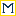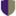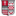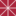Algebraic Geometry Commons™

223 Full-Text Articles 268 Authors 56,862 Downloads69 Institutions

All Articles in Algebraic Geometry

223 full-text articles. Page 8 of 9.

The A-Hypergeometric System Associated With A Monomial Curve, 2011University of Massachusetts - Amherst

The A-Hypergeometric System Associated With A Monomial Curve, E Cattani, C D'Andrea, A Dickenstein

Eduardo Cattani

No abstract provided.

Counting Solutions To Binomial Complete Intersections, 2011University of Massachusetts - Amherst

Counting Solutions To Binomial Complete Intersections, E Cattani, A Dickenstein

Eduardo Cattani

We study the problem of counting the total number of affine solutions of a system of n binomials in n variables over an algebraically closed field of characteristic zero. We show that we may decide in polynomial time if that number is finite. We give a combinatorial formula for computing the total number of affine solutions (with or without multiplicity) from which we deduce that this counting problem is #P-complete. We discuss special cases in which this formula may be computed in polynomial time; in particular, this is true for generic exponent vectors.

Rational Hypergeometric Functions, 2011University of Massachusetts - Amherst

Rational Hypergeometric Functions, E Cattani, A Dickenstein, B Sturmfels

Eduardo Cattani

Multivariate hypergeometric functions associated with toric varieties were introduced by Gel'fand, Kapranov and Zelevinsky. Singularities of such functions are discriminants, that is, divisors projectively dual to torus orbit closures. We show that most of these potential denominators never appear in rational hypergeometric functions. We conjecture that the denominator of any rational hypergeometric function is a product of resultants, that is, a product of special discriminants arising from Cayley configurations. This conjecture is proved for toric hypersurfaces and for toric varieties of dimension at most three. Toric residues are applied to show that every toric resultant appears in the denominator ...

Restriction Of A-Discriminants And Dual Defect Toric Varieties, 2011University of Massachusetts - Amherst

Restriction Of A-Discriminants And Dual Defect Toric Varieties, R Curran, E Cattani

Eduardo Cattani

We study the A-discriminant of toric varieties. We reduce its computation to the case of irreducible configurations and describe its behavior under specialization of some of the variables to zero. We give characterizations of dual defect toric varieties in terms of their Gale dual and classify dual defect toric varieties of codimension less than or equal to four.

Residues In Toric Varieties, 2011University of Massachusetts - Amherst

Residues In Toric Varieties, E Cattani, D Cox, A Dickenstein

Eduardo Cattani

We study residues on a complete toric variety X, which are defined in terms of the homogeneous coordinate ring of X.We first prove a global transformation law for toric residues. When the fan of the toric variety has a simplicial cone of maximal dimension, we can produce an element with toric residue equal to 1. We also show that in certain situations, the toric residue is an isomorphism on an appropriate graded piece of the quotient ring. When X is simplicial, we prove that the toric residue is a sum of local residues. In the case of equal degrees ...

Planar Configurations Of Lattice Vectors And Gkz-Rational Toric Fourfolds In P-6, 2011University of Massachusetts - Amherst

Planar Configurations Of Lattice Vectors And Gkz-Rational Toric Fourfolds In P-6, E Cattani, A Dickenstein

Eduardo Cattani

We introduce a notion of balanced configurations of vectors. This is motivated by the study of rational A-hypergeometric functions in the sense of Gelfand, Kapranov and Zelevinsky. We classify balanced configurations of seven plane vectors up to GL(2,)-equivalence and deduce that the only gkz-rational toric four-folds in 6 are those varieties associated with an essential Cayley configuration. We show that in this case, all rational A-hypergeometric functions may be described in terms of toric residues. This follows from studying a suitable hyperplane arrangement.

The Structure Of Bivariate Rational Hypergeometric Functions, 2011University of Massachusetts - Amherst

The Structure Of Bivariate Rational Hypergeometric Functions, E Cattani, Alicia Dickenstein, Fernando Villegas

Eduardo Cattani

We describe the structure of all codimension-2 lattice configurations A which admit a stable rational A-hypergeometric function, that is a rational function F all the partial derivatives of which are nonzero, and which is a solution of the A-hypergeometric system of partial differential equations defined by Gel′ fand, Kapranov, and Zelevinsky. We show, moreover, that all stable rational A-hypergeometric functions may be described by toric residues and apply our results to study the rationality of bivariate series the coefficients of which are quotients of factorials of linear forms.

Computing Multidimensional Residues, 2011University of Massachusetts - Amherst

Computing Multidimensional Residues, E Cattani, Alicia Dickenstein, Bernd Sturmfels

Eduardo Cattani

Given n polynomials in n variables with a finite number of complex roots, for any of their roots there is a local residue operator assigning a complex number to any polynomial. This is an algebraic, but generally not rational, function of the coefficients. On the other hand, the global residue, which is the sum of the local residues over all roots, depends rationally on the coefficients. This paper deals with symbolic algorithms for evaluating that rational function. Under the assumption that the deformation to the initial forms is flat, for some choice of weights on the variables, we express the ...

Semi-Direct Galois Covers Of The Affine Line, 2011Merrimack College

Semi-Direct Galois Covers Of The Affine Line, Linda Gruendken, Laura L. Hall-Seelig, Bo-Hae Im, Ekin Ozman, Rachel Pries, Katherine Stevenson

Mathematics Faculty Publications

Let k be an algebraically closed field of characteristic p > 0. Let G be a semi-direct product of the form (Z/`Z) b o Z/pZ where b is a positive integer and ` is a prime distinct from p. In this paper, we study Galois covers ψ : Z → P 1 k ramified only over ∞ with Galois group G. We find the minimal genus of a curve Z which admits a covering map of this form and we give an explicit formula for this genus in terms of ` and p. The minimal genus occurs when b equals the order a of ...

2011Harvey Mudd College

Applications Of Convex And Algebraic Geometry To Graphs And Polytopes, Mohamed Omar

All HMC Faculty Publications and Research

No abstract provided.

Strong Nonnegativity And Sums Of Squares On Real Varieties, 2011Harvey Mudd College

Strong Nonnegativity And Sums Of Squares On Real Varieties, Mohamed Omar, Brian Osserman

All HMC Faculty Publications and Research

Motivated by scheme theory, we introduce strong nonnegativity on real varieties, which has the property that a sum of squares is strongly nonnegative. We show that this algebraic property is equivalent to nonnegativity for nonsingular real varieties. Moreover, for singular varieties, we reprove and generalize obstructions of Gouveia and Netzer to the convergence of the theta body hierarchy of convex bodies approximating the convex hull of a real variety.

Complete Graphs Whose Topological Symmetry Groups Are Polyhedral, 2011Pomona College

Complete Graphs Whose Topological Symmetry Groups Are Polyhedral, Erica Flapan, Blake Mellor, Ramin Naimi

Pomona Faculty Publications and Research

We determine for which m the complete graph Km has an embedding in S3 whose topological symmetry group is isomorphic to one of the polyhedral groupsA4, A5 or S4.

A Noneuclidean Universe, 2011Santa Clara University

A Noneuclidean Universe, Frank A. Farris

Mathematics and Computer Science

Let us construct a hypothetical universe, if for no other reason than to challenge our preconceptions about space. We call it a noneuclidean universe because it contradicts some of the notions central to euclidean geometry, where, for instance, the angle measures in a triangle add up to 180 degrees. There are many noneuclidean universes; ours is of a type called hyperbolic. This hypothetical universe has been constructed before. It is often called the Poincaré Upper Halfplane, in honor of French mathematician Henri Poincaré (1854-1912). I admire Poincaré because he is said to have been the last person in the world ...

2011University of Puget Sound

Investigating The Minimal 4-Regular Matchstick Graph, Matt Farley

Summer Research

No abstract provided.

Springer Representations On The Khovanov Springer Varieties, 2011University of Richmond

Springer Representations On The Khovanov Springer Varieties, Heather M. Russell, Julianna Tymoczko

Math and Computer Science Faculty Publications

Springer varieties are studied because their cohomology carries a natural action of the symmetric group Sn and their top-dimensional cohomology is irreducible. In his work on tangle invariants, Khovanov constructed a family of Springer varieties Xn as subvarieties of the product of spheres (S2)n. We show that if Xn is embedded antipodally in (S2)n then the natural Sn-action on (S2)n induces an Sn-representation on the image of H*(Xn). This representation is the Springer representation. Our construction admits an elementary (and geometrically natural) combinatorial description, which we use ...

2011University of Denver

Minimax And Maximin Fitting Of Geometric Objects To Sets Of Points, Yan B. Mayster

Electronic Theses and Dissertations

This thesis addresses several problems in the facility location sub-area of computational geometry. Let S be a set of n points in the plane. We derive algorithms for approximating S by a step function curve of size k < n, i.e., by an x-monotone orthogonal polyline ℜ with k < n horizontal segments. We use the vertical distance to measure the quality of the approximation, i.e., the maximum distance from a point in S to the horizontal segment directly above or below it. We consider two types of problems: min-ε, where the goal is to minimize the error for a ...

The Unimodality Of Pure O-Sequences Of Type Three In Three Variables, 2011Sacred Heart University

The Unimodality Of Pure O-Sequences Of Type Three In Three Variables, Bernadette Boyle

Mathematics Faculty Publications

We will give a positive answer for the unimodality of the Hilbert functions in the smallest open case, that of Artinian level monomial algebras of type three in three variables.

Quaternionic Hermitian Spinor Systems And Compatibility Conditions, 2011Chapman University

Quaternionic Hermitian Spinor Systems And Compatibility Conditions, Alberto Damiano, David Eelbode, Irene Sabadini

Mathematics, Physics, and Computer Science Faculty Articles and Research

In this paper we show that the systems introduced in  and  are equivalent, both giving the notion of quaternionic Hermitian monogenic functions. This makes it possible to prove that the free resolution associated to the system is linear in any dimension, and that the first cohomology module is nontrivial, thus generalizing the results in . Furthermore, exploiting the decomposition of the spinor space into sp(m)-irreducibles, we find a certain number of "algebraic" compatibility conditions for the system, suggesting that the usual spinor reduction is not applicable.

Structures On A K3 Surface, 2010University of Nevada, Las Vegas

Structures On A K3 Surface, Nathan P. Rowe

UNLV Theses, Dissertations, Professional Papers, and Capstones

In the first part of this paper, we examine properties of K3 surfaces of the form:

(x2 + 1)(y2 + 1)(z2 + 1) + Axyz − 2 = 0

We show the surface has Picard number q " 12 by finding 12 curves whose equivalence classes are linearly independent. These curves have self intersection −2. We find the lattice representations of the single-coordinate swapping automorphisms in x, y, and z. We show that we have enough of the Lattice to make accurate predictions of polynomial degree growth under the automorphisms. We describe these automorphisms in terms of operations on elliptic curves.

In the second part ...

2010University of Pennsylvania

The Birational Geometry Of Tropical Compactifications, Colin Diemer

Publicly Accessible Penn Dissertations

We study compactifications of subvarieties of algebraic tori using methods from the still developing subject of tropical geometry. Associated to each ``tropical" compactification is a polyhedral object called a tropical fan. Techniques developed by Hacking, Keel, and Tevelev relate the polyhedral geometry of the tropical variety to the algebraic geometry of the compactification. We compare these constructions to similar classical constructions. The main results of this thesis involve the application of methods from logarithmic geometry in the sense of Iitaka \cite{iitaka} to these compactifications. We derive a precise formula for the log Kodaira dimension and log irregularity in terms ...